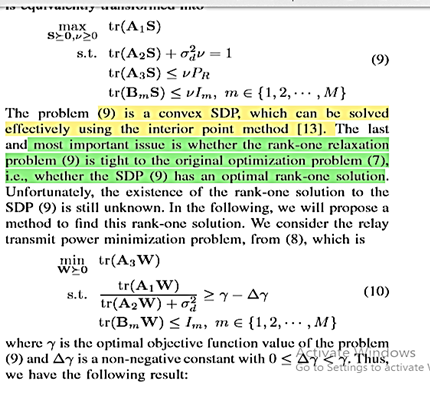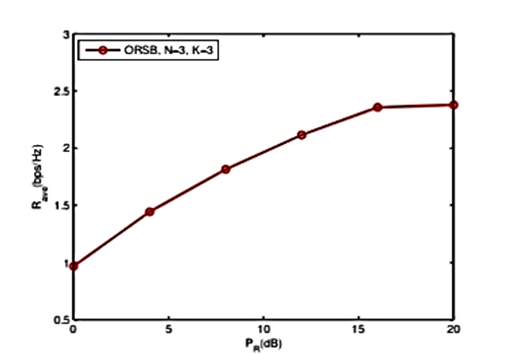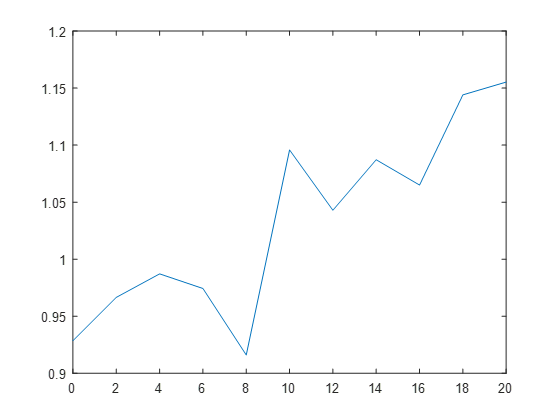# What is wrong with my code?

Hi. I want to solve a convex optimization problem in following article:

[Li, Quanzhong, et al. “Optimal relay selection and beamforming in MIMO cognitive multi-relay networks.” IEEE Communications Letters 17.6 (2013): 1188-1191.]

i have to solve two problems:but the matrices A1,A2,A3,Bm,S and W is not real. (is complex). but all trace(.) are real beacause original problem was real.
but my results arent true. my codes:

``````cvx_begin
variable S(N*N,N*N) hermitian
variable v(1) nonnegative
maximize (real(trace(A11*S)))
subject to
real(trace(A21*S))+sigma2*v==1;
real(trace(A31*S))<=v*PR(ii);
real(trace(B1_1*S))<=v*I1;
real(trace(B2_1*S))<=v*I2;
imag(trace(B2_1*S))==0
imag(trace(A11*S))==0;
imag(trace(B1_1*S))==0;
imag(trace(A31*S))==0;
imag(trace(A21*S))==0;
S==hermitian_semidefinite(N*N);
cvx_end

gamma1=cvx_optval;
cvx_begin
variable W(N*N,N*N) hermitian
minimize (real(trace(A31*W)))
subject to
real(trace(A11*W))-(gamma1-delta_gamma)*real(trace(A21*W))-(gamma1-delta_gamma)*sigma2>=0;
real(B1_1*W)<=I1;
real(B2_1*W)<=I2;
imag(B2_1*W)==0;
imag(B1_1*W)==0;
imag(trace(A11*W))==0;
imag(trace(A21*W))==0;
imag(trace(A31*W))==0;
W==hermitian_semidefinite(N*N);
cvx_end
``````

You haven’t provided input data. Therefore, forum readers can’t reproduce your results, whatever they are.

but my results arent true.

What specifically is "not true? Please show the solver and CVX output from running your programs. If something is “not true”, please explain what is not true, and show the evidence supporting that. For instance, the values of optimal variables or any MATLAB calculations performed with the optimal values after CVX execution.

Please keep in mind that the solvers employed by CVX allow for slight infeasibilities, e.g., of order 1e-8 in constraints at a returned optimum. For instance the minimum eigenvalue of a Hermitian semidefinite matrix may be slightly negative, for example, -1e-8. Because of roundoff, expressions which are real in exact arithmetic, may have a roundoff level imaginary term.

thanks, I have to plot the following curve( after 1000 iteration and calculating average of optimal values,besides i have to solve problem 9 and 10 for three nodes.(k=3) but i have provided for one of them in last post, the other are the same.),but my curve is very different. ( I will send it).
and W is not rank one matrix. ( according to the article ,solution of problem 10 is rank one matrix).

input data:

N=3;% number of antenna for each relay

M=2;% number of primary users. there is one secondary transmitter(SU_Tx) and one secondary reciever(SU_RX)

PS=10;% maximum allowable transmit power of the SU_Tx

sigma2=1;% covariance matrix of Additive White Gaussian Noise(AWGN) @ k’th relay is: “sigma2*I”

PR_dB=0:2:20;% maximum allowable transmit power of the relay
PR=10exp(0.1PR_dB);
I1=sigma2;%Interfrence Threshold on PU_1 reciever
I2=sigma2;%Interfrence Threshold on PU_2 reciever

g1=(1/sqrt(2))(randn(M,1)+1irandn(M,1));
g2=(1/sqrt(2))(randn(N,M)+1irandn(N,M));

h_1k=(1/sqrt(2))(randn(N,k)+1irandn(N,k));
h_2k=(1/sqrt(2))(randn(N,k)+1irandn(N,k));

h1=kron(conj(h_1k(:,1)),h_2k(:,1));
H11=kron(conj(h_1k(:,1)),eye(N));
H21=kron(eye(N),h_2k(:,1))

G11_1=kron(conj(h_1k(:,1)),g2(:,1));
G12_1=kron(conj(h_1k(:,1)),g2(:,2));
G21=kron(eye(N),g2(:,1));
G22=kron(eye(N),g2(:,2));

p1=I1./(abs(g1(1,1))^2);
p2=I2./(abs(g1(2,1))^2);
ps=min(PS,min(p1,p2));

A11=psh1(h1)’;
A21=sigma2H21(H21)’
A31=psH11(H11)’+sigma2eye(NN,N*N);

B1_1=psG11_1(G11_1)’+sigma2G21(G21)’;
B2_1=psG12_1(G12_1)’+sigma2G22(G22)’

my result!!!( after 100 repeat)The text in the image states for optimization problem (9) that the existence of rank one solution is unknown. So why are you complaining about not getting a rank one solution?. Even if it were known that there exists an optimal solution that is rank one, then unless it there is a unique optimal solution, there would be no guarantee that the optimal solution you obtain would be rank one even if someone else found an optimal solution which is rank one,

A rank one constraint is non-convex. So not every convex relaxation of a problem having a rank one constraint will have every optimal solution, or maybe even any optimal solution, be rank one.

Getting a rank one solution is apparently what (10) and whatever is about. if you have specific concerns about the results in the paper, then after doing some more checking yourself, perhaps you should email the authors of the paper. I know nothing about the paper or the authors, but I will point out that not all published papers, even in prestigious refereed journals, are free of substantive errors.

There is nothing wrong with separately specifying equality constraints for real and imaginary parts as you did, but it can be done with a single equality constraint, as discussed at http://cvxr.com/cvx/doc/dcp.html#constraints

One or both sides of an equality constraint may be complex; inequality constraints, on the other hand, must be real. A complex equality constraint is equivalent to two real equality constraints, one for the real part and one for the imaginary part. An equality constraint with a real side and a complex side has the effect of constraining the imaginary part of the complex side to be zero.